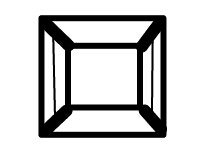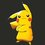# RMO 2014 (Rajasthan region)

1. Let ABCD be an isosceles trapezium having an incircle; let AB and CD be the parallel sides and let CE be the perpendicular from C on to AB. Prove that AB, CCE and CD are in GP.

2. If $x$ and $y$ are positive real numbers, prove that $4x^{4}+4y^{3}+5x^{2}+y+1\geq 12xy$.

3. Determine all pairs $m$>$n$ of positive integers such that $1=gcd(n+1,m+1)=gcd(n+2,m+2)=......=gcd(m,2m-n)$

4. What is the minimal area of right-angled triangle whose inradius is 1 unit?

5. Let ABC be an acute-angled triangle and let I be its incentre. Let the incircle of triangle ABC touch BC in D. The incircle of the triangle ABD touches AB in E, the incircle of triangle ACD touches BC in F. Prove that B, E, I, F are concyclic.

6. In the adjacent figure, can the numbers 1,2,3,...,18 be placed, one on each line segment such that the sum of the numbers on the three line segments meeting at each point is divisible by 3.Image Question 6Note by Pranjal Jain
6 years, 8 months ago

This discussion board is a place to discuss our Daily Challenges and the math and science related to those challenges. Explanations are more than just a solution — they should explain the steps and thinking strategies that you used to obtain the solution. Comments should further the discussion of math and science.

When posting on Brilliant:

• Use the emojis to react to an explanation, whether you're congratulating a job well done , or just really confused .
• Ask specific questions about the challenge or the steps in somebody's explanation. Well-posed questions can add a lot to the discussion, but posting "I don't understand!" doesn't help anyone.
• Try to contribute something new to the discussion, whether it is an extension, generalization or other idea related to the challenge.

MarkdownAppears as
*italics* or _italics_ italics
**bold** or __bold__ bold
- bulleted- list
• bulleted
• list
1. numbered2. list
1. numbered
2. list
Note: you must add a full line of space before and after lists for them to show up correctly
paragraph 1paragraph 2

paragraph 1

paragraph 2

[example link](https://brilliant.org)example link
> This is a quote
This is a quote
    # I indented these lines
# 4 spaces, and now they show
# up as a code block.

print "hello world"
# I indented these lines
# 4 spaces, and now they show
# up as a code block.

print "hello world"
MathAppears as
Remember to wrap math in $$ ... $$ or $ ... $ to ensure proper formatting.
2 \times 3 $2 \times 3$
2^{34} $2^{34}$
a_{i-1} $a_{i-1}$
\frac{2}{3} $\frac{2}{3}$
\sqrt{2} $\sqrt{2}$
\sum_{i=1}^3 $\sum_{i=1}^3$
\sin \theta $\sin \theta$
\boxed{123} $\boxed{123}$

Sort by:

(2). By AM-GM, $4y^{3}+y \geq 4y^{2}, 4x^{4}+1 \geq 4x^{2}, 4x^{2}+5x^{2}+4y^{2}=9x^{2}+4y^{2} \geq 12xy$.

This is using the fact that for all positive $a, b, a+b \geq 2\sqrt {ab}$.

- 6 years, 8 months ago

OM*G. This was so easily simplified by you :( :( :( :( ...I did 30-40% of the same using AM-GM...... Felt that the powers were disproportionately large and left it midway :/ :'( (sob)

- 6 years, 8 months ago

Never! I gave RMO 2014, Rajasthan region. Paper was completely different but the number of questions. Why?

- 6 years, 8 months ago

I got the same paper. :D How many did you solve? (How can you appear when in 12th, BTW?)

- 6 years, 8 months ago

I didnt appeard! My brother did! I took paper frm him!

- 6 years, 7 months ago

Oh, I see :P. Which class is he in? How many did he do?

- 6 years, 7 months ago

He is in 9th. Attempted RMO just for some exposure. I didn't asked him how much he did as I know he won't tell anyone!

- 6 years, 7 months ago

-No Issues- :)

- 6 years, 7 months ago

How much are you scoring in NSEs?

- 6 years, 7 months ago

I didn't write them this year because I had to write another test on that day. Anyway, I wasn't prepared upto 12th level in any of the subjects :/ , So there wasn't any point in giving them. What about you?

- 6 years, 7 months ago

NSEC=180, NSEA=144, NSEP=105

- 6 years, 7 months ago

Impressive !!!!!!!!! :D

- 6 years, 7 months ago

Not at all!! NSEC was worth 200+, No comments about astro and NSEP changed its pattern. I was prepared for subjective ones. Well just waiting for results. Just some hopes in NSEC and NSEA.

- 6 years, 7 months ago

(3). Note that gcd (m-n, n+i)=1, i=1, 2, ..., m-n. Otherwise, gcd (m-n+n+i, n+i)=gcd (m+i, n+i) > 1. But one of n+i must be a multiple of m-n. Let it be n+x. Then gcd(n+x, m+x)= (n+x, m-n) is at least m-n. So m-n=1.

Note that gcd (n+1, m+1)=gcd (n+1, n+2)=1. Hence all pairs are in the form (x, x+1) for an integer x.

- 6 years, 7 months ago

For number 4, The minimum area of right triangle is (3 - 2 sqrt(2)) square units.

Using the relationship of inradius, semiperimeter, and area of triangles, by letting a and b be the sides of the triangle and c be the hypotenuse, (a + b + c)/2 = ab/2 implying the relationship. By Pythagorean theorem, a + b - ab = -sqrt(a^2 + b^2). After manipulations, it implies that ab + 2 = 2b + 2a. We solve for a in terms of b, where a = (2b - 2)/(b - 2) and ab/2 = (2b^2 - 2b)/2(b - 2). I am trying to get a non-calculus solution here, but using derivatives, the minimum area is the given answer above...

P.S. Somehow confused because the function relating the area and one side has actually no minimum as graphed... What might the actual answer be?

- 6 years, 7 months ago

It's 3+2 root 2. Not -

- 6 years, 7 months ago

Calculus is not allowed in RMO.

- 6 years, 7 months ago

FYI, it is allowed but not required. All the sums can be solved without calculus. However, if one wants to use calculus, one is allowed to do so.

- 6 years, 7 months ago

Well, I really didn't knew this! I still doubt it...

- 6 years, 7 months ago

I got the answer as 3-2sqrt2 without using calculus. We'll get a quadratic equation in the side and hence the area taking both sides as equal. I got the minimum side as 2-sqrt2

- 5 years, 8 months ago